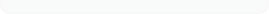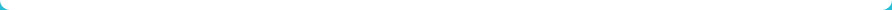Custom Search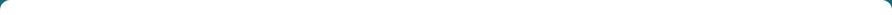Featured Services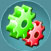Tutorials on Embedded
System Design .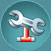Tutorials on
Antenna Design.Tutorials on
Image Processing Using
Open-CV.# PROJECT IDEAS

HELP SHARE IDEAS.COM
Mail us at : info@helpshareideas.com

:Uploaded on Thursday 27th OCT 2016

## DESIGN OF DUAL BAND MICRO-STRIP PATCH ANTENNA FOR WIRELESS APPLICATIONS.

In this article, We are going to discuss the procedure for designing a rectangular Microstrip patch antenna and then we will explain How a Dualband rectangular Micro-strip patch antenna can be designed for use in wireless applications.

#### Design Specifications for a Micro-strip Patch Antenna

A Micro-strip Patch Antenna essentially will have a Substrate material made of some Di-Electric material with ground plane. Below structure depicts the typical Micro-strip Patch Antenna structure. We are not going to discuss on the feeding techniques and other basics of Antenna Design as it will be covered in separate module in Tutorial Section.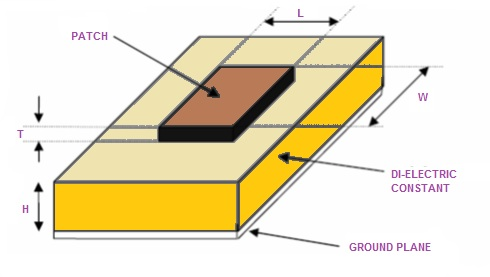##### The three essential parameters for the design of a rectangular Microstrip Patch Antenna are:

• Frequency of operation (fo ):
• The resonant frequency of the antenna must be selected appropriately. The Personal Communication System (PCS) uses the frequency range from 1850-1990 MHz. Hence the antenna designed must be able to operate in this frequency range. The resonant frequency selected for the design currently discussing is 2.4 GHz.
• Dielectric constant of the substrate (er ):
• The dielectric material selected for the current design is FR4_epoxy which has a dielectric constant of 4.4. A substrate with a high dielectric constant has been selected since it reduces the dimensions of the antenna.
• Height of dielectric substrate ( h ):
• For the microstrip patch antenna to be used in wireless applications, it is essential that the antenna is not bulky. Hence, the height of the dielectric substrate is selected as 1.6 mm.
Hence, the essential parameters for the current Dual Band Micro-Strip Patch Antenna design are:
• f0 = 2.4 GHz
• er= 4.4
• h = 1.6 mm

#### How to Calculate the Parameters

##### Step 1: Calculation of the Width (W ):

The width of the Microstrip patch antenna is given by equation shown in the below image.Substituting c = 3e8 m/s, e r = 4.4 and f o = 2.4GHz, we get: W = 0.0380363=38.0363mm.

##### Step 2: Calculation of Effective dielectric constant (e reff ):

Below equation gives the effective dielectric constant as: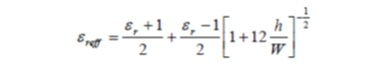Substituting er = 4.4, W = 38.0363 mm and h = 1.6 mm we get: er eff = 4.0858.

##### Step 3: Calculation of the Effective length (L eff ):

Below equation gives the effective length as follows:Substituting er eff = 4.0858, c = 3e8 m/s and f o = 2.4 GHz we get: L eff = .03092=30.920mm.

##### Step 4: Calculation of the length extension (Delta L):

Below equation is used to calculate the Length Extension.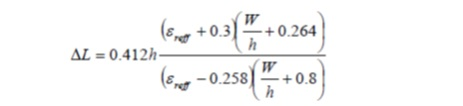Substituting e reff =4.0858 , W = 38.0363 mm and h = 1.6 mm we get: Delta L = .7388mm.

##### Step 5: Calculation of actual length of patch ( L ):

Below equation is used to calculate The actual length which is obtained by re-writing the above equation .Substituting L eff = 30.920 mm and Delta L = .7388 mm we get: L = .0294425=29.4425mm.

##### Step 6: Calculation of the ground plane dimensions ( Lg and Wg ):

The transmission line model is applicable to infinite ground planes only. However, for practical considerations, it is essential to have a finite ground plane. It has been shown by  that similar results for finite and infinite ground plane can be obtained if the size of the ground plane is greater than the patch dimensions by approximately six times the substrate thickness all around the periphery. Hence, for this design, the ground plane dimensions would be given as

Lg=6h+L=6(1.6mm)+29.4425mm=.03904=39.04mm=40mm.
Wg=6h+W=6(1.6mm)+38.0363mm=.0476=47.63mm=48mm.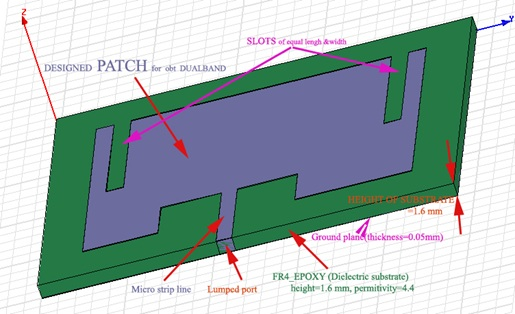#### Structure of the Dual Band Micro-strip Patch Antenna with Dimensions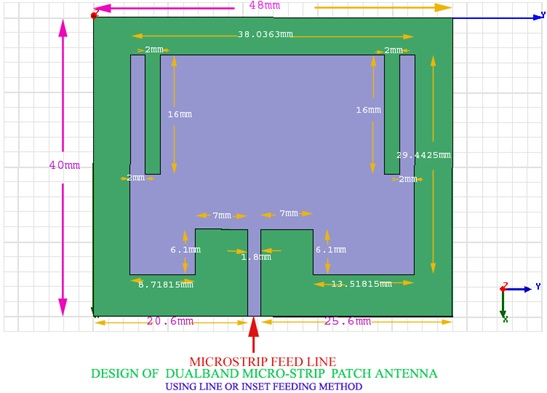##### Editor: HelpShareIdeas.comResearch on Academic ProjectsTo assist the students we upload some of the Implemented Projects with code Students make use of it by downloading the same. Watch the Site for more details Kindly Visit again. Read moreEmbedded PROJECTs with CodeKeep checking the Site for more details Help Share Ideas Website is still under Beta Version.